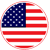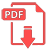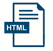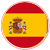# Home | Mathematics | Exponents and Radicals

## Simplifying Exponential Expressions

Basic rules for exponentiation

If n is a positive integer and x is any real number, then xn corresponds to repeated multiplication
x5= x*×*x*×*×*x
We can call this “x raised to the power of 5,” “x to the power of 5,” or simply “x to the 5.” Here, x is the base and 5 is the exponent or the power.

From this definition, we can deduce some basic rules that exponentiation must follow as well as some hand special cases that follow from the rules.

The rules and special cases are summarized in the following table. Below, we give details for each one.
Download pdf file:
Author: Carlos Utrera

Paper size:EnglishSpanishCopyright © 2013     EduGnosis.com  | About US    All Rights Reserved.